# Three Phase Switched Reluctance Machine

Description of the Three Phase Switched Reluctance Machine component in Schematic Editor.

Table 1. Three Phase Switched Reluctance Machine
component component dialog window component parameters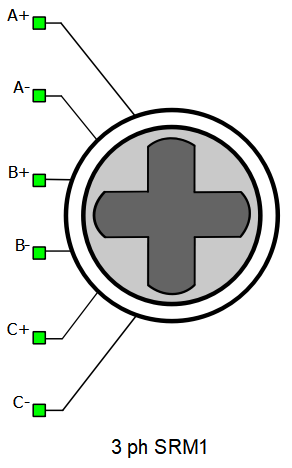• Property tabs:
A+, A-, B+, B-, C+, and C- are terminals of stator A, B, and C windings, respectively. Each of the stator windings uses the single phase variant of the current source interface.

## Electrical sub-system model

The electrical part of the machine is represented by the following system of equations.

$\left[\begin{array}{c}{v}_{\alpha s}\\ {v}_{bs}\\ \begin{array}{c}{v}_{cs}^{}\\ \end{array}\end{array}\right]=\left[\begin{array}{ccc}{R}_{s}& 0& 0\\ 0& {R}_{s}& 0\\ 0& 0& {R}_{s}^{}\end{array}\right]\left[\begin{array}{c}{i}_{as}\\ {i}_{bs}\\ \begin{array}{c}{i}_{cs}^{}\\ \end{array}\end{array}\right]+\frac{d}{dt}\left[\begin{array}{c}{\psi }_{as}\\ {\psi }_{bs}\\ \begin{array}{c}{\psi }_{cs}^{}\\ \end{array}\end{array}\right]$

where:

${T}_{e}={T}_{ea}+{T}_{eb}+{T}_{ec}$

where:

Table 2. Three Phase Switched Reluctance Machine electrical subsystem model variables
symbol description
ψas Stator phase A flux [Wb]
ψbs Stator phase B flux [Wb]
ψcs Stator phase C flux [Wb]
ias Stator phase A current [A]
ibs Stator phase B current [A]
ics Stator phase C current [A]
vas Stator phase A voltage[V]
vbs Stator phase B voltage[V]
vcs Stator phase C voltage[V]
Rs Stator phase resistance [Ω]
Te Machine developed electromagnetic torque [Nm]
Tea Machine developed electromagnetic torque in phase a [Nm]
Teb Machine developed electromagnetic torque in phase b [Nm]
Tec Machine developed electromagnetic torque in phase c [Nm]

## Mechanical sub-system model

Motion equation:

Table 3. Mechanical sub-system model variables
symbol description
Jm Combined rotor and load moment of inertia [kgm2]
Te Machine developed electromagnetic torque [Nm]
Tl Shaft mechanical load torque [Nm]
b Machine viscous friction coefficient [Nms]
Note: Motion equation is the same for all of the rotating machine models.

## Electrical

Table 4. Electrical parameters
symbol description
Rs Stator phase resistance [Ω]
Ns Number of stator poles
Nr Number of rotor poles
theta vector Mechanical angle input vector [deg]
current vector Stator phase current input vector [A]
current table Stator phase current lookup table [A]
Te table Electrical torque lookup table [Nm]
The nonlinear characteristics of the switched reluctance machine are represented using lookup tables. The model can be parametrized by providing either:
• Flux linkage and torque lookup tables, or
• Stator current and torque lookup tables.
The flux linkage and torque lookup tables should be functions of the machine mechanical angle and the corresponding phase's stator current, while the current lookup table should be a function of the machine mechanical angle and the corresponding phase's flux linkage. The input vectors (theta vector, current vector, flux linkage vector) must be monotonically increasing and the number of elements in each of them must match the number of elements along the corresponding dimension in the provided lookup table. The lookup tables use linear interpolation and linear extrapolation.

The lookup tables should be provided either as nested Python lists or 2-dimensional numpy arrays. For the flux linkage and the torque tables, the numbers of elements in the inner lists must match the number of elements in the stator current vector, while the number of elements in the outer list must match the number of elements in the machine mechanical angle vector. For the stator current table, the numbers of elements in the inner lists must match the number of elements in the flux linkage vector, while the number of elements in the outer list must match the number of elements in the machine mechanical angle vector. This is summarized in Figure 1, Figure 2, and Figure 3.

## Mechanical

Table 5. Mechanical parameters
symbol description
Nr The number of rotor teeth
Jm Combined rotor and load moment of inertia [kgm2]
Friction coefficient Machine viscous friction coefficient [Nms]
Unconstrained mechanical angle Limiting mechanical angle between 0 and 2π

symbol description
Load source Load can be set from SCADA/external or from model (in model case, one signal processing input will appear)
Load ai offset Assigned offset value to the input signal representing external torque command
Load ai gain Assigned gain value to the input signal representing external torque command

External load enables you to use an analog input signal from a HIL analog channel with the load_ai_pin address as an external torque/speed load, and to assign offset (V) and gain (Nm/V) to the input signal, according to the formula:

${T}_{l}=load_ai_gain·\left(AI\left(load_ai_pin\right)+load_ai_offset\right)$

Note: An analog input pin may be overwritten if another component uses the same analog input pin. If another property (from the same or a different component) uses the same analog input pin, the input signal value will be applied to only one of those properties. For instance, if both the load and the resolver carrier signal use the same analog input pin, the signal value will only be applied only to one of these.

## Feedback

Table 7. Feedback parameters
symbol description
Encoder ppr Incremental encoder number of pulses per revolution
Encoder Z pulse length Z digital signal pulse length in periods. Can be Quarter length or Full period (default)
Resolver pole pairs Resolver number of pole pairs
Resolver carrier source Resolver carrier signal source selection (internal or external)
External resolver carrier source type External resolver carrier signal source type selection (single ended or differential); available only if the Resolver carrier source property is set to external
Resolver carrier frequency Resolver carrier signal frequency (internal carrier) [Hz]
Resolver ai pin 1 Resolver carrier input channel 1 address (external carrier)
Resolver ai pin 2 Resolver carrier input channel 2 address (external carrier); available only if the External resolver carrier source type property is set to differential
Resolver ai offset Resolver carrier input channel offset (external carrier)
Resolver ai gain Resolver carrier input channel gain (external carrier)
Absolute encoder protocol Standardized protocol providing the absolute machine encoder position

If an external resolver carrier source is selected, the source signal type can be set as either single ended or differential. The single ended external resolver carrier source type enables use of an analog input signal from the HIL analog channel with the res_ai_pin_1 address as the external carrier source. Additionally, offset (V) and gain (V/V) values can be assigned to the input signal, according to the formula:

$res_carr_src=res_ai_gain·\left(AI\left(res_ai_pin_1\right)+res_ai_offset\right)$

The differential external resolver carrier source type enables use of two analog input signals from the HIL analog channels with the res_ai_pin_1 and the res_ai_pin_2 addresses. Analog signals from these HIL analog inputs are subtracted, and the resulting signal is used as the external differential carrier source. Additionally, offset (V) and gain (V/V) values can be assigned to the input signal (similarly to the single ended case), according to the formula:

$res_carr_src=res_ai_gain·\left(\left(AI\left(res_ai_pin_1\right)-AI\left(res_ai_pin_2\right)\right)+res_ai_offset\right)$
Note: Differential external resolver signal support is available from the 2023.1 software version. Only the single ended external resolver option was available in previous software versions.
Note: In order to get a resolver signal with an amplitude of 1 when using an external carrier signal, the offset and the gain should be chosen in such a way that the resolver carrier signal has an amplitude of 1 after the adjustment. As shown in Figure 1, the sinusoidal signal used to generate external resolver carrier source is fed to HIL analog input 1. The analog input signal is scaled in order to get the resolver signals with an amplitude of 1.
Note: An analog input pin may be overwritten if another component uses the same analog input pin. If another property (from the same or a different component) uses the same analog input pin, the input signal value will be applied to only one of those properties. E.g. if both the load and the resolver carrier signal use the same analog input pin, the signal value will be applied only to one of these.

The following expression must hold in order to properly generate the encoder signals:

$4·enc_ppr·{f}_{m}{·T}_{s}\le 1$
Table 8. Variables in the encoder limitation expression
symbol description
enc_ppr Encoder number of pulses per revolution
fm Rotor mechanical frequency [Hz]
Ts Simulation time step [s]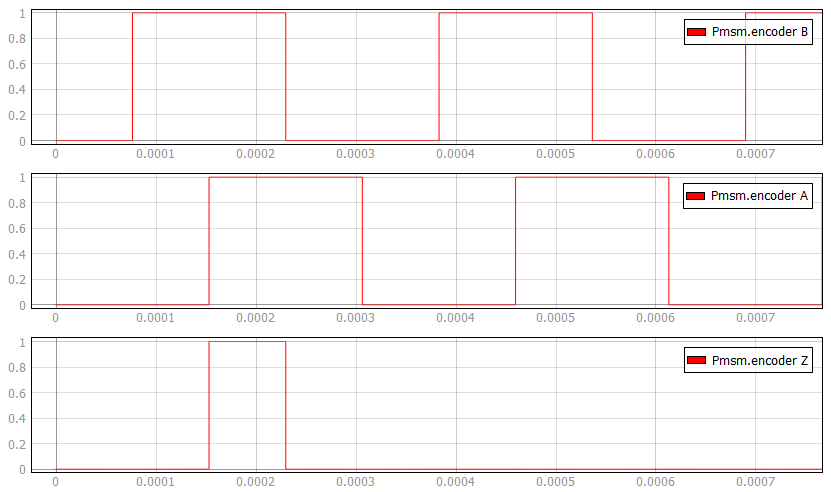Figure 5. Machine encoder signals when Full period option is selected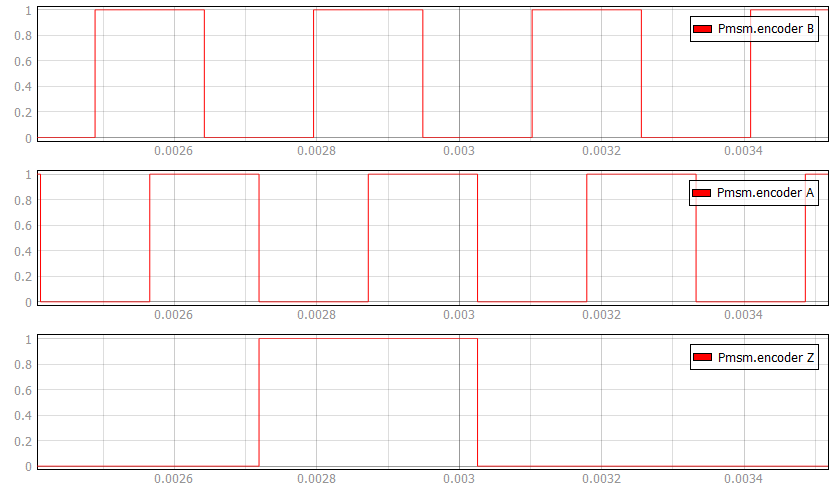Figure 6. Machine encoder signals when Quarter period option is selected
Note: While the machine speed is positive, the encoder channel B signal leads the encoder channel A signal.
Note: Absolute encoder protocol is not supported on HIL402 (configurations: 1, 2, 3, and 4).

## Snubber

All machines with current source based circuit interfaces have the Snubber tab in the properties window where the value of snubber resistance can be set. Snubbers are necessary in the cases when an inverter or a contactor is directly connected to the machine terminals. This value can be set to infinite (inf), but it is not recommended when a machine is directly connected to the inverter since there will be a current source directly connected to an open switch. In this case, one of each switch pairs S1 and S2, S3 and S4, and S5 and S6 will be forced closed by the circuit solver in order to avoid the topological conflicts. On the other hand, with finite snubber values, there's always a path for the currents Ia and Ib, so all inverter switches can be open in this case. Circuit representations of this circuit without and with snubber resistors are shown in Figure 4 and Figure 5 respectively. Snubbers are connected across the current sources.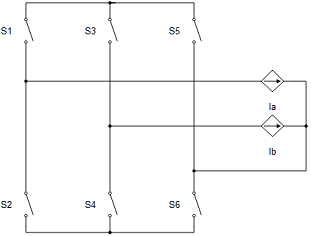Figure 7. Circuit representation of machine and inverter when all the switches are open without resistors (not recommended)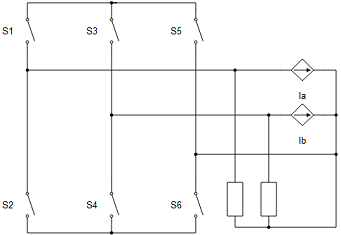Figure 8. Circuit representation of machine and inverter when all the switches are open with resistors (recommended)
Note: Snubbers exist only in the machine components that have the current source based circuit interface.
Note: Snubbers are dynamic, which means that the snubber is dynamically added to circuit modes where topological conflicts are detected.
Table 9. Snubber parameters
symbol description
Rsnb stator Stator snubber resistance value [Ω]

## Output

This block tab enables a single, vectorized signal output from the machine. The output vector contains selected machine mechanical and/or electrical variables in the same order as listed in this tab.

Note: All machine components have the Execution rate, Electrical torque, Mechanical speed, and Mechanical angle, but the rest of the signals differ from component to component.
Table 10. Output parameters
symbol description
Execution rate Signal processing output execution rate [s]
Electrical torque Machine electrical torque [Nm]
Mechanical speed Machine mechanical angular speed [rad/s]
Mechanical angle Machine mechanical angle [rad]
Stator phase A flux Stator phase A magnetic flux [Wb]
Stator phase B flux Stator phase B magnetic flux [Wb]
Stator phase C flux Stator phase C magnetic flux [Wb]
Phase a torque component Electric torque developed in phase a [Nm]
Phase b torque component Electric torque developed in phase b [Nm]
Phase c torque component Electric torque developed in phase c [Nm]